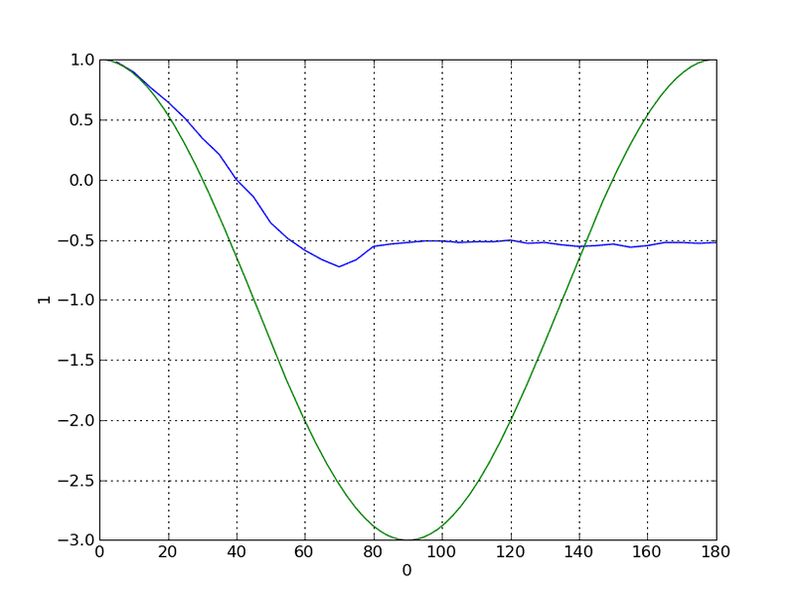# Drag on a Cylinder - Confused

## Homework Statement

I'm writing a lab report on drag on a non-rotating cylinder. The drag coefficient is calculated using the Pressure co-efficient. The problem I'm facing is that my lab states that the pressure on the cylinder can be predicted by $$\left(p-p_{0}\right)_{max} \times cos(\theta)$$

## The Attempt at a Solution

The research I've done on the subject leads me to conclude that the pressure on a cylinder can be predicted by $$\left(p-p_{0}\right)_{max} \times \left(1-4sin^{2}(\theta)$$ instead of the above mentioned equation.

The following is a plot I did in pylab with the experimental plot in blue and the therotical plot in green (using the 2nd equation). The y-axis represents the coeffiecent of pressure while the x-axis is the position from the stagnation point on the cylinder, in degrees.My question is, am I missing something? Or is my lab wrong on this? I've been literally reading every material I can get my hands on for the past 3 or 4 hours and I'm still terribly confused.

EDIT: The following is the text from my lab notebook which confuses me.

Drag acts in the plane of motion so the pressure acting in this plane on an element at θ degrees to the cylinder is (p-p0)cosθ. The area it acts upon is small and equal to Rδθ for a unit length of the cylinder. The force on the element in the plane of the drag is the pressure multiplied by the area of the element = (p - p0)Rcosθδθ.

Last edited:

"Drag acts in the plane of motion so the pressure acting in this plane on an element at θ degrees to the cylinder is (p-p0)cosθ."

The wording might be a bit confusing.

It should read something like: The contribution of pressure to Drag is (p-p0) cosθ at an angle θ. Since force is calculated by the following equation

F = - INTEGRAL (p * n )dS , wheren is the normal vector.

it is easy to see that the contribution to drag (Fx: force in the x-direction) is in fact (p-p0) cosθ. (simply by using Cartesian coordinates)

**sorry, i dont know how to add all the fancy math symbols.

following through your calculations you should get
[text]\left(p-p_{0}\right)_{max} \times \left(1-4sin^{2}(\theta)[/tex]

"The force on the element in the plane of the drag is the pressure multiplied by the area of the element = (p - p0)Rcosθδθ."

basically the equation i wrote above for force, but this is for a differential force (dFx).

hopefully this was of some use.

I'm sorry,
this is wrong:

\left(p-p_{0}\right)_{max} \times \left(1-4sin^{2}(\theta)

you should solve for p-p0 through Bernoulli's, but the rest i wrote should be okay.

To get 1-4sin^2(x), I did use Bernoulli's. The following page gives the derivation, you'll have to scroll down a bit.

http://www.ecourses.ou.edu/cgi-bin/ebook.cgi?doc=&topic=fl&chap_sec=07.4&page=theory

Since,

$$p_{0}+\frac{1}{2}\rho U^{2} = p + \frac{1}{2}\rho v^{2}_{\theta}$$

where U is the velocity of the air flow and v is the velocity of the airflow at $$\theta$$. v is given by v = $$2U\sin\theta$$

Rearranging for p-p0

$$p-p_{0} = \frac{1}{2}\rho\left(U^{2} - v^{2}_{\theta}\right)}$$

Subtituting into the equation for Cp:

$$C_{p} = \frac{\frac{1}{2}\rho\left(U^{2} - v^{2}_{\theta}\right)}{\frac{1}{2}\rho U^{2}}$$

If I substitute $$v = 2U\sin\theta$$ in the above equation and cancel various terms, I end up with the following:

$$C_{p} = 1 - 4\sin^{2}\theta$$

Does this make sense? I have not studied this material into this much depth, I knew Bernoulli but I don't know how we end up with $$v = 2U\sin\theta$$, so I just took it on faith from the above mentioned website and did the derivation.

I just want to show a theoretical pressure distribution i.e pressure distribution around a cylinder in a ideal flow, thats why I'm hunting for a function (and ended up with the above mentioned one) for Cp in terms of the position of the cylinder.

your equations are correct. It seems to me that if you read the section "Flow Past a Fixed Circular Cylinder" in the link you provided, you will know how v = 2 U sin(theta)